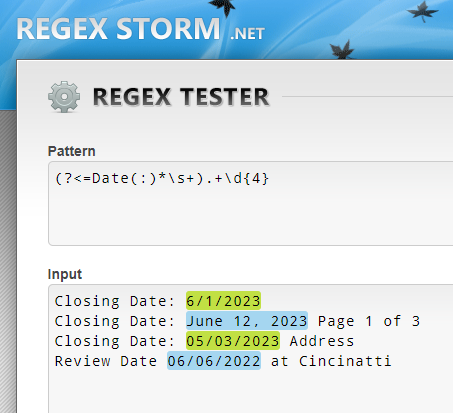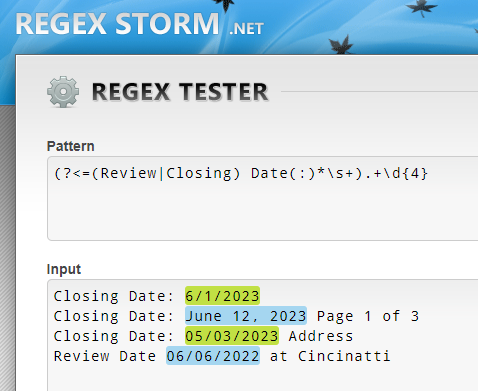# Modify regex

Hi the following regular expression is getting every thing after Closing Date:
“(?<=Closing Date:).*”
But some time there is some text after the date. The date can be in several formats. I need to get only the date and not the text after that. How can I change the regex?

Thanks a lot

@A_Learner give me example

Closing Date: 6/1/2023
Closing Date: June 12, 2023 Page 1 of 3
Review Date 06/06/2022 at Cincinatti

@A_Learner
Try this one:

``````(?<=Closing Date: )[A-Za-z]{3,9} \d{1,2}, \d{4}|(?<=Closing Date: )\d{1,2}\/\d{1,2}\/\d{4}|(?<=Review Date: )\d{1,2}\/\d{1,2}\/\d{4}
``````

Option 1:
(?<=Date(:)*\s+).+\d{4}.

.
Option 2: